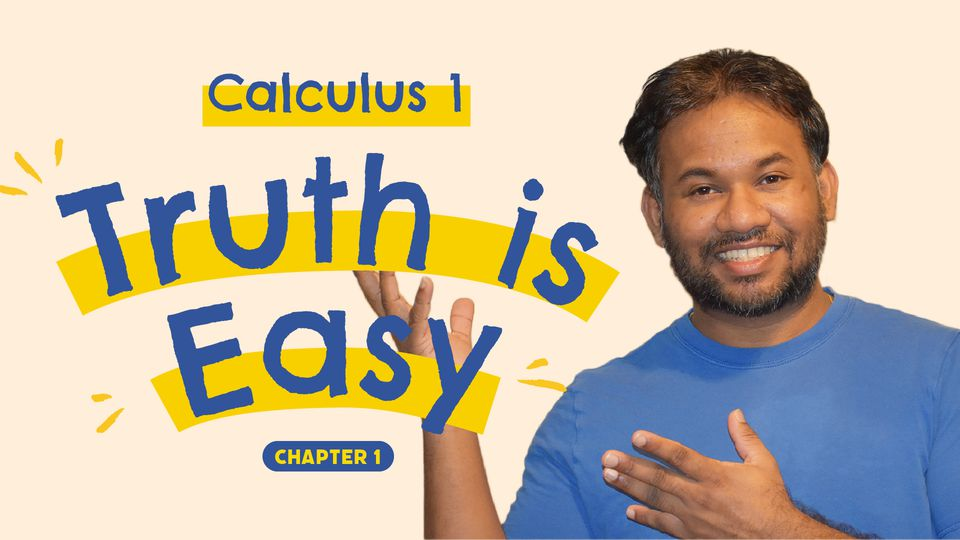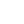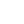Preview the Course
43m

# Calculus 1, Chapter 1: Part 2 - Preliminaries

by Miyuran D. | Math

7 Lessons 43:14
1.1. Intro 43
2.2. Absolute Value 2:13
3.3. Examples 14:01
4.4. Distance in Two Dimensions 2:29
5.5. Equation of a Circle 6:40
6.6. Midpoint Formular 1:04
7.7. Trigonometry 16:04

43m

## Overview

Here in this section, we present a review of fundamental concepts such as absolute value function, some basic geometry concepts such as distance in two dimensions, equation of a circle, and midpoint formula. Finally, we talk about trigonometry functions that we will use throughout this course.

Calculus is the mathematics of change in motion. Calculus is used to model phenomena that change. Calculus was developed independently in the late 17th century by Isaac Newton and Gottfried Wilhelm Leibniz. Calculus II is all about integral calculus.

Mainly in this course, we are learning a bunch of techniques to solve many different problems with applications. The main topics of calculus we're going to discuss are going to be finding limits: talk about what happens eventually, derivatives: instantaneous rate of change, and integrals: which talk about the area.

This course is with five main chapters:

1 Preliminaries
2. Limit and continuity
3. Differentiation
4. Application of Derivatives.
5. Integration.

Tags:

• Calculus
• Derivatives
• Functions
• Graphs
• Logarithms
• Math
• STEM

## Instructor

I am Dr. Miuran Dencil, a professional mathematician currently working as an assistant professor. I earned my master’s and doctoral degrees in mathematics in 2016 and 2020 from Texas Tech University, Texas, USA. While in college, I won very prestigious Presidential Graduate Fellowship for 5 years, which is offered to very best graduate student in the school. I was invited speaker for many recognized conferences and won many grants for my research. My dissertation research was Geometric Properties of Special Functions and Related Quadratic Differentials. My research interests include Partial Differential Equations and Number Theory. I graduated from University of Kelaniya, Sri Lanka in 2011 with Bachelor's Degree, which is special degree in mathematics with first class honors.

Teaching is my passion and calling! on top of that mathematics is fun and interactive! I started to teach mathematics at very young age. I have over 16 years of teaching experiences and taught mathematics to kindergarten through senior high school students, college level and graduate level classes.
Once I graduated from my bachelor’s degree, I worked as an instructor at University of Kelaniya from 2011-2013, then worked as a lecturer at university of moratuwa from 2013-2015. Then I started my graduate studies at Texas Tech University while working as GPTI/ Research assistant 2015-2020.
I have taught: Calculus I, II, III + Pre-Cal, AP Cal AB/ AP Cal BC, Trigonometry, Linear Algebra, Geometry, ODE/PDE, Algebra 1,2, Numerical Methods, Real/ Advanced Analysis, Complex Numbers, Discrete Mathematics, Group Theory/ Ring Theory/Number Theory, Integration/ Laplace equations/ Fourier Series- Transformation, Grade Mathematics, Mechanics/ Motion of a particle/ Force- equilibrium/ Harmonic motion, Trainer for exams such as SAT, GRE math sections.

Over 16 years of my teaching experiences and long academic life I have found techniques which helped me to understand and solve any given problem faster and accurate. Now I am ready to teach them to you as I believe nothing is too challenging as long as the student is willing to learn.

As a mathematics is interactive and fun subject it allow students to get extra practice and provide personalized learning atmosphere for students to sharpened their analytical thinking and problem solving technique.

See full profile